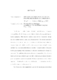## Discrete Optimization Methods for Segmentation and Matching2012
##### Authors
Liu, Ming-Yu
This dissertation studies discrete optimization methods for several computer vision problems. In the first part, a new objective function for superpixel segmentation is proposed. This objective function consists of two components: entropy rate of a random walk on a graph and a balancing term. The entropy rate favors formation of compact and homogeneous clusters, while the balancing function encourages clusters with similar sizes. I present a new graph construction for images and show that this construction induces a matroid. The segmentation is then given by the graph topology which maximizes the objective function under the matroid constraint. By exploiting submodular and monotonic properties of the objective function, I develop an efficient algorithm with a worst-case performance bound of $\frac{1}{2}$ for the superpixel segmentation problem. Extensive experiments on the Berkeley segmentation benchmark show the proposed algorithm outperforms the state of the art in all the standard evaluation metrics. Next, I propose a video segmentation algorithm by maximizing a submodular objective function subject to a matroid constraint. This function is similar to the standard energy function in computer vision with unary terms, pairwise terms from the Potts model, and a novel higher-order term based on appearance histograms. I show that the standard Potts model prior, which becomes non-submodular for multi-label problems, still induces a submodular function in a maximization framework. A new higher-order prior further enforces consistency in the appearance histograms both spatially and temporally across the video. The matroid constraint leads to a simple algorithm with a performance bound of $\frac{1}{2}$. A branch and bound procedure is also presented to improve the solution computed by the algorithm. The last part of the dissertation studies the object localization problem in images given a single hand-drawn example or a gallery of shapes as the object model. Although many shape matching algorithms have been proposed for the problem, chamfer matching remains to be the preferred method when speed and robustness are considered. In this dissertation, I significantly improve the accuracy of chamfer matching while reducing the computational time from linear to sublinear (shown empirically). It is achieved by incorporating edge orientation information in the matching algorithm so the resulting cost function is piecewise smooth and the cost variation is tightly bounded. Moreover, I present a sublinear time algorithm for exact computation of the directional chamfer matching score using techniques from 3D distance transforms and directional integral images. In addition, the smooth cost function allows one to bound the cost distribution of large neighborhoods and skip the bad hypotheses. Experiments show that the proposed approach improves the speed of the original chamfer matching up to an order of 45 times, and it is much faster than many state of art techniques while the accuracy is comparable. I further demonstrate the application of the proposed algorithm in providing seamless operation for a robotic bin picking system.